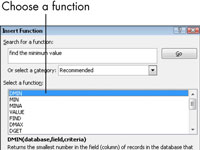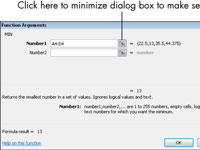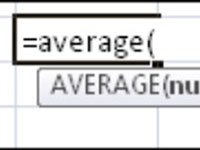How to Understand Excel Functions - dummies

Sometimes, writing an Excel formula to perform an Excel calculation is awkward or lengthy. An Excel function refers to a certain math calculation. Excel functions can greatly shortcut the amount of typing you have to do to create a particular result.

For example, suppose that you want to sum the values in cells A1 through A10. To express it as a formula, you’d have to write out each cell reference individually: =A1+A2+A3+A4+A5+A6+A7+A8+A9+A10

For example, instead of the using the preceding formula, you could sum, using the Excel SUM function: =SUM(A1:A10)

With an Excel function, you can represent a range with the upper-left corner’s cell reference, a colon, and the lower-right corner’s cell reference. In the case of A1:A10, there is only one column, so the upper left is A1 and the lower right is A10.

Each Excel function has one or more arguments. An argument is a placeholder for a number, text string, or cell reference. For example, the SUM function requires at least one argument: a range of cells. So in the preceding example, A1:A10 is the argument. The arguments for a function are enclosed in a set of parentheses.

Each Excel function has its own rules as to how many required and optional arguments it has, and what they represent. You don’t have to memorize the sequence of arguments (the syntax) for each function; Excel asks you for them. It can even suggest a function to use for a certain situation if you aren’t sure what you need.

To find a function and get help with its syntax, follow these steps:

1Click in the cell where you want to insert the function. Choose Formulas→Insert Function.

The Insert Function dialog box opens.2Find the function you want to insert.

If you don’t know what function you want, type a few keywords (in the Search for a Function field) that represent what you want to do. For example, if you want to find the minimum value in a range of cells, you might type Find the minimum value. Then click Go to see a list of functions that might be what you want. Click each function on the list and read the description of it that appears.3When you find the function you want, click OK.

The Function Arguments dialog box.

4Fill in the arguments in the field provided.

The arguments will be different depending on the function you choose. You can fill in the arguments by typing a number or a cell reference (or range) directly into the box. Or you can click the Selector button to the right of the text box, which collapses the dialog box temporarily. Select the cell or range in the worksheet and then press Enter to return to the dialog box.5Click OK.

After you get comfortable with a particular function, you may prefer to type it directly into the cell rather than going through the Insert Function dialog box. As you type, Excel helps you by providing prompts for the arguments in a ScreenTip.